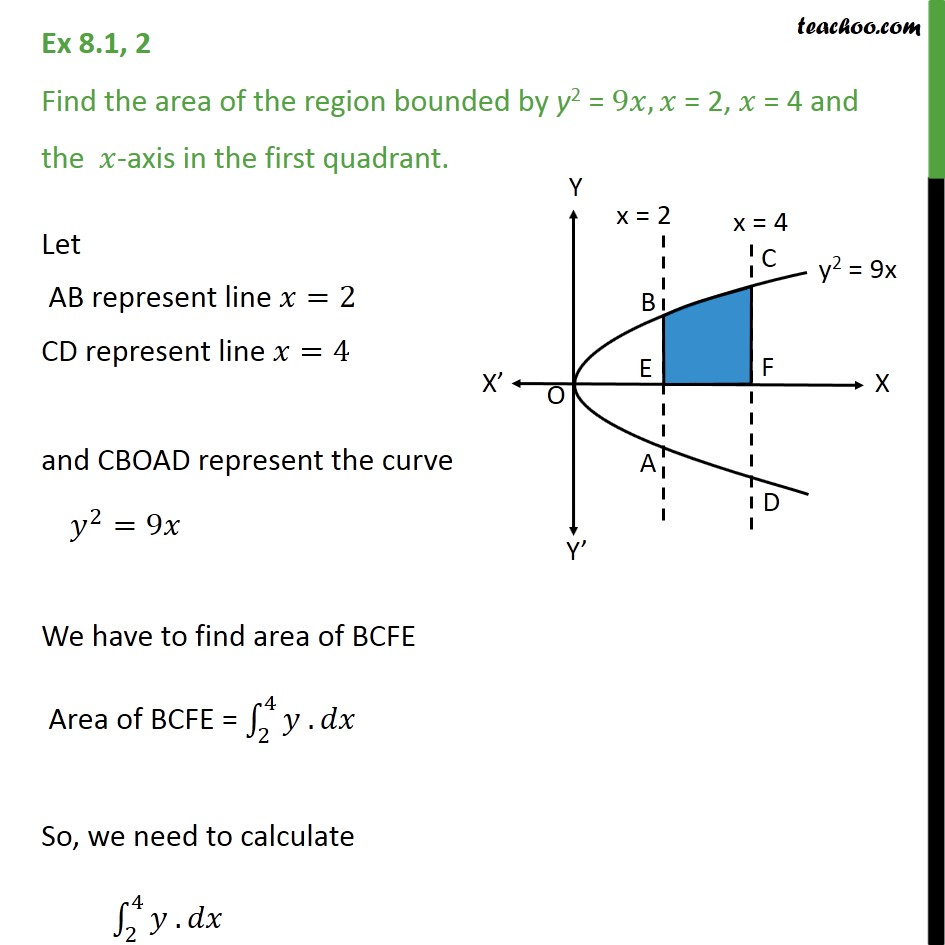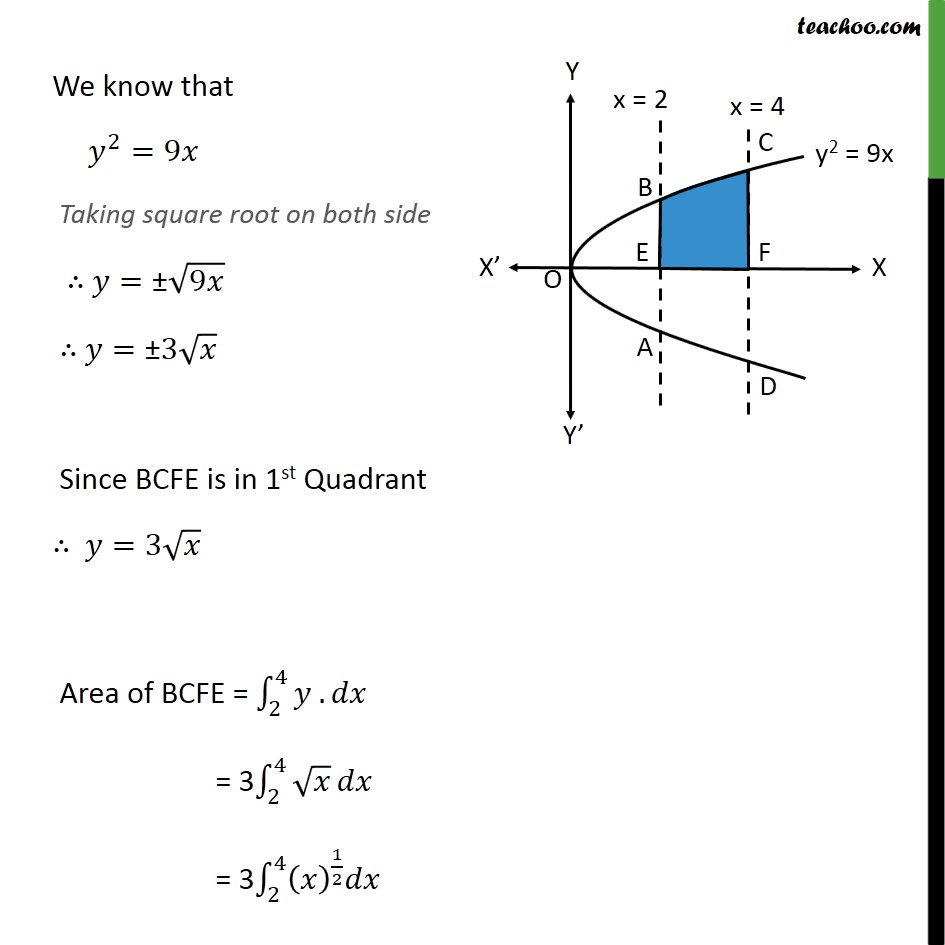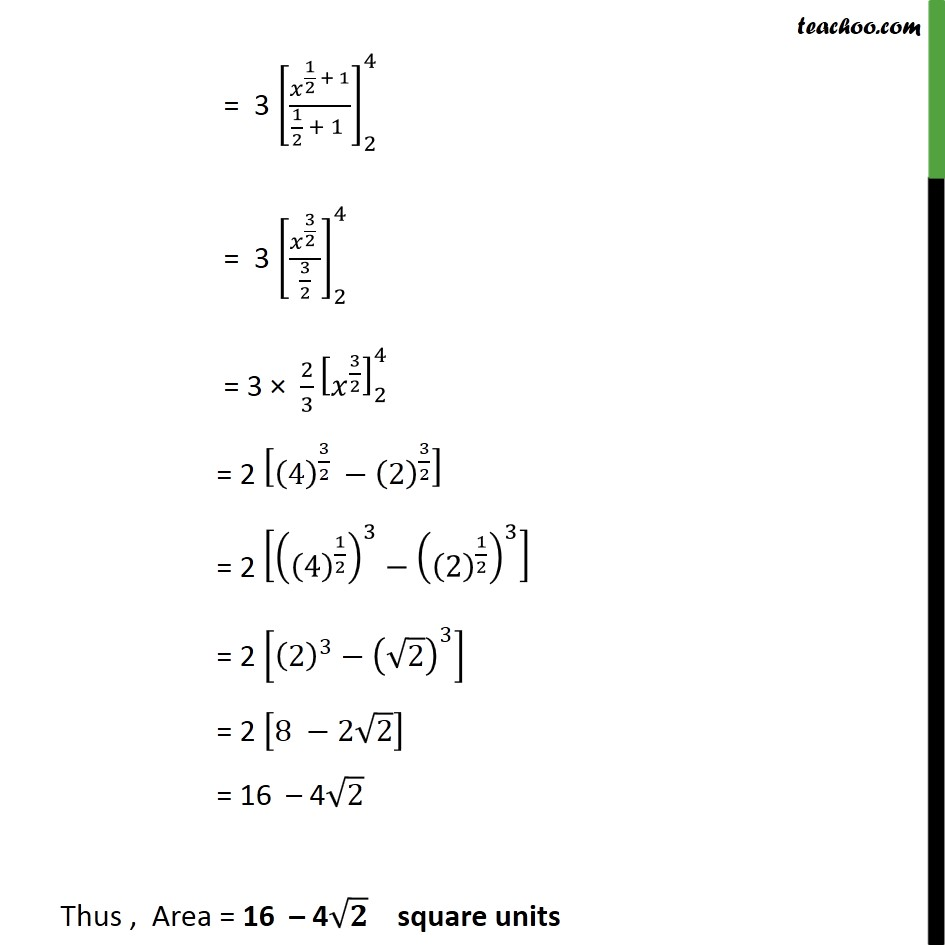1. Chapter 8 Class 12 Application of Integrals
2. Serial order wise
3. Ex 8.1

Transcript

Ex 8.1, 2 Find the area of the region bounded by y2 = 9𝑥, 𝑥 = 2, 𝑥 = 4 and the 𝑥-axis in the first quadrant. Let AB represent line 𝑥=2 CD represent line 𝑥=4 and CBOAD represent the curve 𝑦﷮2﷯=9𝑥 We have to find area of BCFE Area of BCFE = 2﷮4﷮𝑦 .﷯𝑑𝑥 So, we need to calculate 2﷮4﷮𝑦 .﷯𝑑𝑥 We know that 𝑦﷮2﷯=9𝑥 Taking square root on both side ∴ 𝑦=± ﷮9𝑥﷯ ∴ 𝑦=±3 ﷮𝑥﷯ Since BCFE is in 1st Quadrant ∴ 𝑦=3 ﷮𝑥﷯ Area of BCFE = 2﷮4﷮𝑦 .﷯𝑑𝑥 = 3 2﷮4﷮ ﷮𝑥﷯﷯𝑑𝑥 = 3 2﷮4﷮ 𝑥﷯﷮ 1﷮2﷯﷯𝑑𝑥﷯ = 3 𝑥﷮ 1﷮2﷯ + 1﷯﷮ 1﷮2﷯ + 1 ﷯﷯﷮2﷮4﷯ = 3 𝑥﷮ 3﷮2﷯ ﷯﷮ 3﷮2﷯﷯﷯﷮2﷮4﷯ = 3 × 2﷮3﷯ 𝑥﷮ 3﷮2﷯﷯﷯﷮2﷮4﷯ = 2 4﷯﷮ 3﷮2﷯ ﷯− 2﷯﷮ 3﷮2﷯﷯﷯ = 2 4﷯﷮ 1﷮2﷯﷯﷯﷮3﷯− 2﷯﷮ 1﷮2﷯﷯﷯﷮3﷯﷯ = 2 2﷯﷮3﷯− ﷮2﷯﷯﷮3﷯﷯ = 2 8 −2 ﷮2﷯﷯ = 16 – 4 ﷮2﷯ Thus , Area = 16 – 4 ﷮𝟐﷯ square units

Ex 8.1